Next: Loading Up: Convergence criteria Previous: Network iterations   Contents

### Implicit dynamics

In CalculiX, implicit dynamics is implemented using the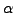-method . The method is unconditionally stable and second order accurate. The parameter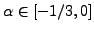represents high frequency damping. The lower the value, the more high frequency dissipation is introduced. This is frequently desired in order to reduce noice. However, it also leads to energy loss.

An analysis has shown that the usual static convergence criteria have to be supplemented by energy criteria in order to obtain good results. To this end, the relative energy balance is used defined by: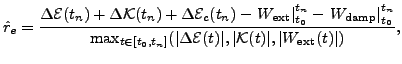(456)

where

•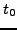is the time at the beginning of the present step
•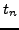is the actual step time
•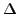denotes the difference of a quantity between the actual time and the time at the beginning of the step
•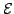is the internal energy
•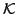is the kinetic energy
•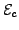is the contact spring energy
•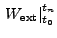is the external work done since the start of the present step
•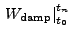is the work done by damping since the start of the present step (always negative)
• in the denominator the choice of the kinetic energy versus the CHANGE of the internal energy is on purpose.

At the start of the step the relative energy balance is zero. During the step it usually decreases (becomes negative) and increases in size. Limiting the relative energy decay at the end of the step to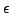, during the step the following minimum energy decay function is proposed: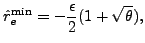(457)

where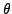is the relative step time,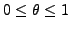. The following algorithm is now used:

If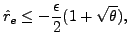(458)

the increment size is decreased. Else if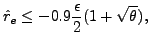(459)

the increment size is kept. Else it is increased.

In dynamic calculations contact is frequently an important issue. As soon as more than one body is modeled they may and generally will come into contact. In CalculiX penalty contact is implemented by the use of springs, either in a node-to-face version or in a face-to-face version. A detailed analysis of contact phenomena in dynamic calculations  has revealed that there are three instances at which energy may be lost: at the time of impact, during persistent contact and at the time of rebound.

At the time of impact a relative energy decrease has been observed, whereas at the time of rebound a relative energy increase occurs. The reason for this is the finite time increment during which impact or rebound takes place. During closed contact the contact forces do not perform any work (they are equal and opposite and are subject to a common motion). However, in the increment during which impact or rebound occurs, they do perform work in the part of the increment during which the gap is not closed. The more precise the time of impact coincides with the beginning or end of an increment, the smaller the error. Therefore, the following convergence criteria are prososed:

At impact the relative energy decrease (after impact minus before impact) should not exceed 0.008, i.e.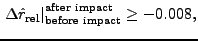(460)

else the increment size is decreased by a factor of 4.

At rebound the relative energy increase between the time of rebound and the time of impact should not exceed 0.0025, i.e.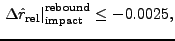(461)

else the increment size is decreased by a factor of 2.

In between impact and rebound (persistent contact) both the impact criterion as well as the rebound criterion has to be satisfied. Furthermore it has been observed that during contact frequently vibrations are generated corresponding to the eigenfrequency of the contact springs. Due to the high frequency damping characteristics of the-method this contributes additionally to a decay of the relative energy. To avoid this, the time increment should ideally exceed the period of these oscillations substantionally,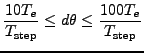(462)

is aimed at, where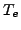is the period of the oscillations,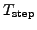is the duration of the step and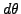is the relative increment size.Next: Loading Up: Convergence criteria Previous: Network iterations   Contents
guido dhondt 2018-12-15